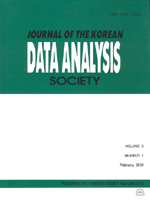상세검색
최근 검색어 전체 삭제
다국어입력
즐겨찾기0KCI등재 학술저널

# Some Probability Range of Confidence Intervals on the Linear Combinations of Variances

• 등재여부 : KCI등재
• 2002.06
• 155 - 164 (10 pages)

The confidence intervals for variance A of the ratio of total variance in the two-factor nested variance component model with equal numbers in the cells is given by various forms by many authors. This article shows that some of these forms of probability ranges of Wang s and Broemeling s confidence intervals for variance A of total variance are equals and whether grater than 1- α or not in the two-factor nested variance component model.

1. Introduction

2. Variance component Model

3. The Range of Confidence Intervals

References